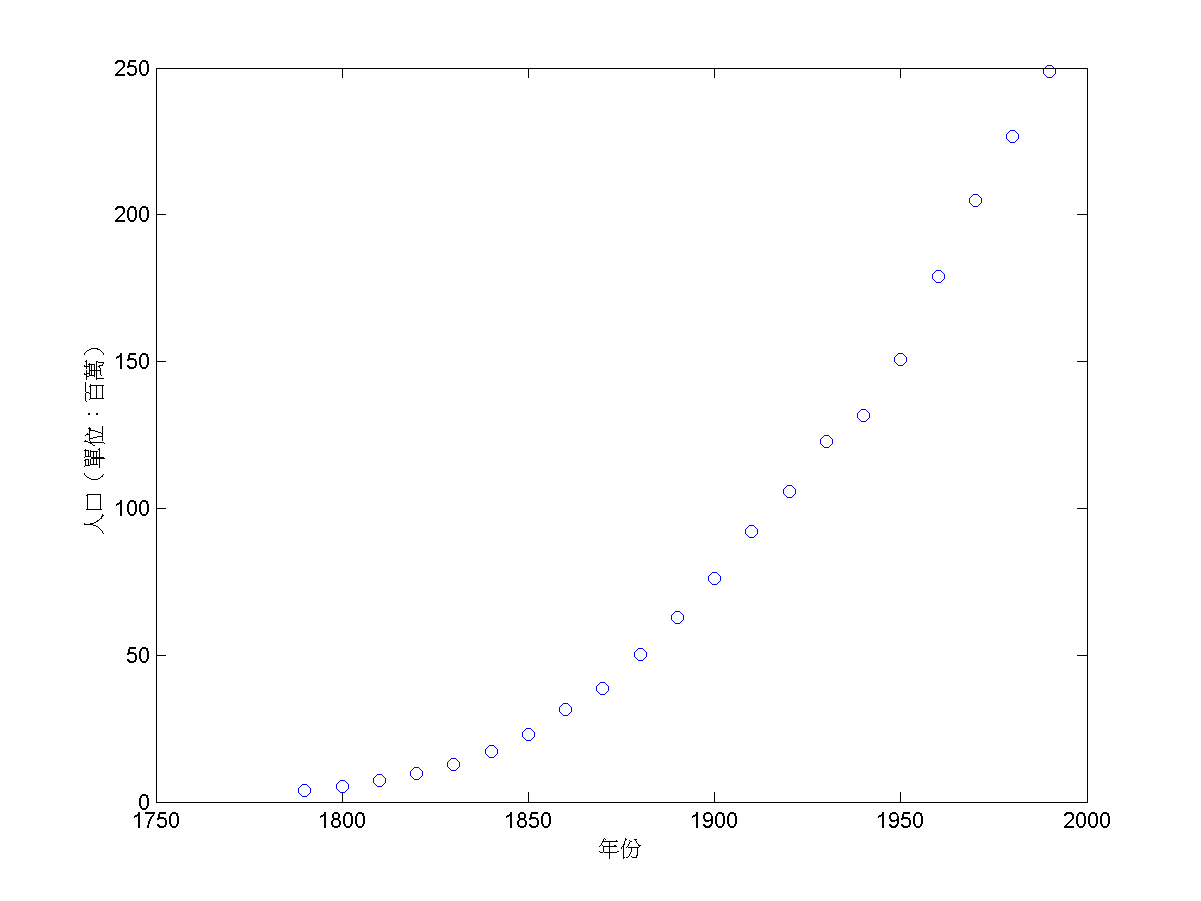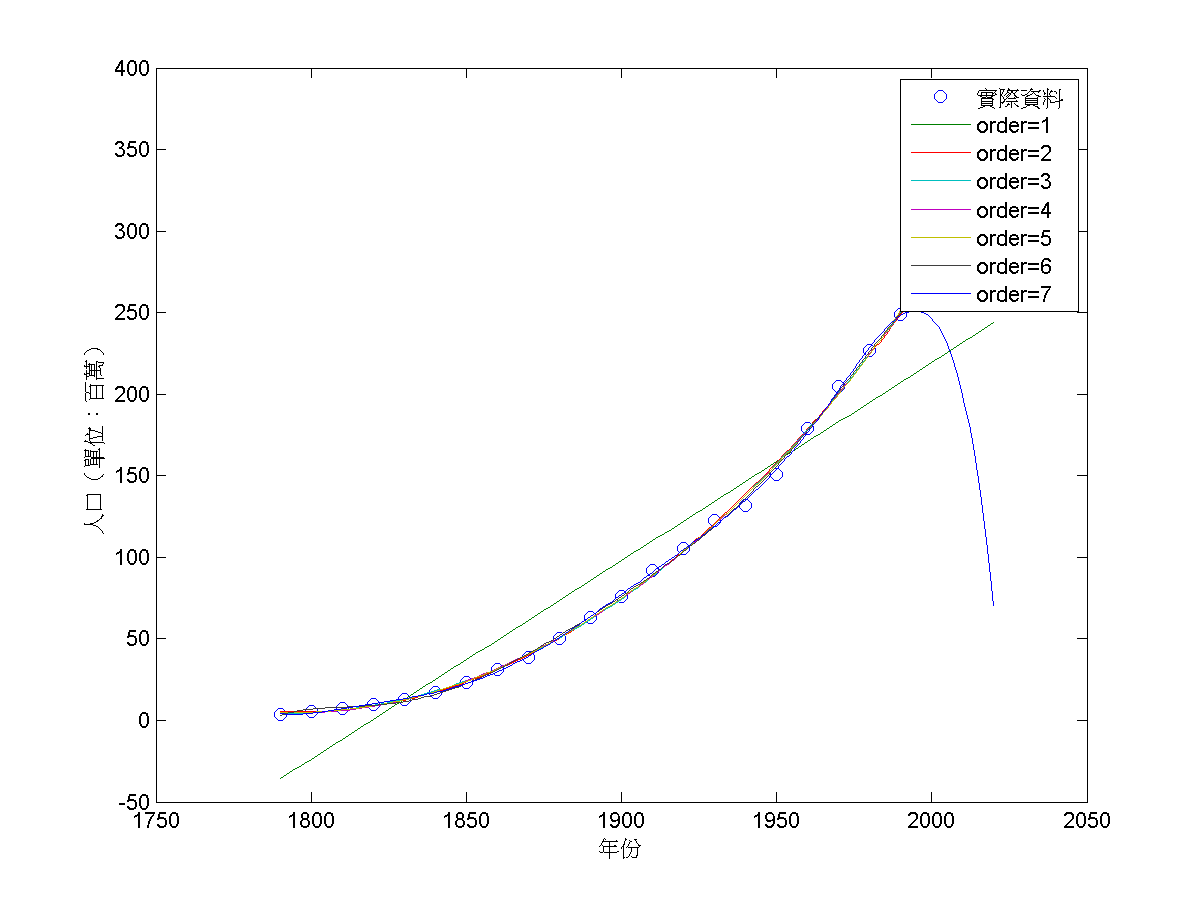## 7-5 憭𡁻?撘𤩺挱??

Example 1: 07-多項式的處理與分析/plotCensus.mload census.mat plot(cdate, pop, 'o'); xlabel('年份'); ylabel('人口（單位：百萬）');Example 2: 07-多項式的處理與分析/polyfit01.mwarning off % 刪除此列將可顯示警告訊息 load census.mat plot(cdate, pop, 'o'); p3 = polyfit(cdate, pop, 3); cdate2 = 1790:2002; pop2 = polyval(p3, cdate2); plot(cdate, pop, 'o', cdate2, pop2, '-'); xlabel('年份'); ylabel('人口（單位：百萬）'); legend('實際人口', '預測人口'); popAt2002 = polyval(p3, 2002)popAt2002 = 282.5332Example 3: 07-多項式的處理與分析/polyfit02.mwarning off % 刪除此列將可顯示警告訊息 load census.mat cdate2 = min(cdate):(max(cdate)+30); curve = zeros(7, length(cdate2)); for i = 1:7 curve(i,:) = polyval(polyfit(cdate,pop,i), cdate2); end plot(cdate, pop,'o', cdate2, curve); legend('實際資料', 'order=1', 'order=2','order=3', 'order=4', 'order=5', 'order=6', 'order=7'); xlabel('年份'); ylabel('人口（單位：百萬）');Hint

MATLAB程式設計：進階篇